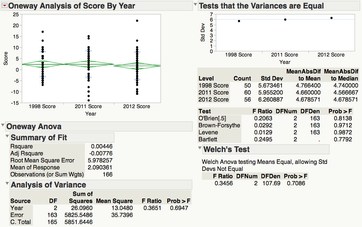## Backgammon Case Study

The Backgammon case study and data sets.

One-Way ANOVA:

Determine if a backgammon program is about the same in 2011 and 2012 as it was in 1998, or if there appear to have been upgrades at some point. Also, determine whether a professor is demonstrably superior in play to the program.  Key ideas: One-Way ANOVA, testing assumptions, F ratio, R2, and basic computations.

JMP features demonstrated:

Analyze > Distribution, Tables > Stack, Analyze > Fit Y by X, Formula Editor

Statistical/Graphical Tools Used:

Histograms, confidence intervals, stacking data, One-Way ANOVA, Unequal Variances test, one-sample t-Test, ANOVA table and calculations, F Distribution, F ratios.Revision History:

5/3/2016:  Fixed an error in the formula on Page 12.  The denominator was changed from n-1 to k-1.  Thanks for a student at the UC San Diego for pointing this out.

Statistics case studies provided by Dewayne Derryberry of the Math Department at Idaho State University.